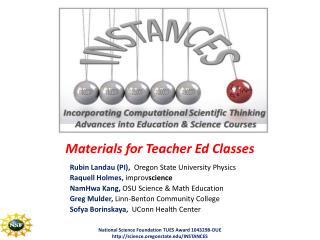DownloadDownload PresentationMaterials for Teacher Ed Classes

# Materials for Teacher Ed Classes

Download Presentation## Materials for Teacher Ed Classes

- - - - - - - - - - - - - - - - - - - - - - - - - - - E N D - - - - - - - - - - - - - - - - - - - - - - - - - - -
##### Presentation Transcript

1. Materials for Teacher Ed Classes Rubin Landau (PI), Oregon State University Physics Raquell Holmes, improvscience NamHwa Kang, OSU Science & Math Education Greg Mulder, Linn-Benton Community College SofyaBorinskaya, UConn Health Center National Science Foundation TUES Award 1043298-DUE http://science.oregonstate.edu/INSTANCES

2. Motivations • Computational Science view: CS + math + science • Computation is essential in science • Simulation part of scientific process • To change K-16, change Teacher Education • A single CS class not enough • Need ability to look inside application black box • Improved pedagogy via problem-solving context INSTANCES, XSEDE13

3. Motivations For Pre- & In-Service Teachers • Science + scientific process • Include simulation, data & math • Complexity via simplicity • Numerical & analytic solutions • Data via computing Aim: teach computing use as part of science Disciplines: physics, biology INSTANCES, XSEDE13

4. Module Content • Teacher Materials • Learning objectives • Model validations • CST goals, objectives • Background readings • Student Reading (culled) • Exercises • “Programming” • Implementations • Excel, Python, Vensim INSTANCES, XSEDE13

5. Classroom Context Schools of Education • Science and Math Ed 412-413 • Pre-service teachers • Technology-Inquiry in Math and Science • 1 week instruction • Post survey • Computational Physics Modules taught • Spontaneous Decay & Bugs (exponential growth) • Excel, VenSim, Python • Student Readings • Exercises INSTANCES, XSEDE13

6. SED 412-413 • 20 PTs, 14 respondents • 1 previous CS course • Most excel • No python or vensim • Additional comments • Length of time to learn application • Extensive background material provided Feedback from 20 PTs on their perceptions of the applications INSTANCES, XSEDE13

7. Module Collection • Computer Precision • Spontaneous Decay • Biological Growth • Bug Population Dynamics • Random Numbers • Random Walk • Projectiles + Drag • Trial & Error Search INSTANCES, XSEDE13

8. E.G.: Limits and Precision Limits in Excel Limits.py • Computational Science Thinking • Computers = experimental lab • Computers = finite • Range: natural, compute numbers • → Floating pt numbers ǂ exact • Student exercises Limits in Python INSTANCES, XSEDE13

9. E.G.: Limits and Precision Precision in Excel • CST • Computers = experimental lab • Computers = finite • Range: natural, compute numbers • → Floating pt numbers ǂ exact • Student exercises VenSim INSTANCES, XSEDE13

10. E.G.: Random Numbers • Introduce chance into computing • Stochastic natural processes • CST • Pseudo-random numbers • Need look, check numbers INSTANCES, XSEDE13

11. E.G.: 3-D Random Walks 3D Walk.py Perfume diffusion Brownian motion INSTANCES, XSEDE13

12. E.G.: Spontaneous Decay Simulation Algorithm: if random < , decay • CST • Sounds like Geiger (real world)? • How know what’s real? • Real meaning of simulation • Meaning of exponential decay DecaySound.py INSTANCES, XSEDE13 INSTANCES, XSEDE13

13. E.G.: Stone Throwing Integration • CST • New way to do math (stochastic) • Calculus via experiment • Rejection technique INSTANCES, XSEDE13 INSTANCES, XSEDE13

14. Conclusions • Group challenge: level of math, of science • Early Assessment • scientific process helps • balance: background vs exercises • disparity computing backgrounds • need literacy + programming tool • Truer Effectiveness: need full course • Help! - Need science educator replacement, • Biology examples INSTANCES, XSEDE13

15. K-12 Standards “Understand numbers, ways of representing numbers, relationships among numbers, and number systems; Understand patterns, relations, and functions; Use mathematical models to represent and understand quantitative relationships; Use visualization, spatial reasoning, and geometric modeling to solve problems” ~Principles and Standards for School Mathematics, National Council of Teachers of Mathematics, 2000. INSTANCES, XSEDE13